Gas Laws

 Boyle's Law Amonton's Law Charles' Law Gay-Lussac's Law Avogadro's Hypothesis The Ideal Gas Equation Ideal Gas Calculations Dalton's Law of Partial Pressures

Boyle's Law

Torricelli's experiment did more than just show that air has weight; it also provided a way of creating a vacuum because the space above the column of mercury at the top of a barometer is almost completely empty. (It is free of air or other gases except a negligible amount of mercury vapor.) Torricelli's work with a vacuum soon caught the eye of the British scientist Robert Boyle.

Boyle's most famous experiments with gases dealt with what he called the "spring of air." These experiments were based on the observation that gases are elastic. (They return to their original size and shape after being stretched or squeezed.) Boyle studied the elasticity of gases in a J-tube similar to the apparatus shown in the figure below. By adding mercury to the open end of the tube, he trapped a small volume of air in the sealed end.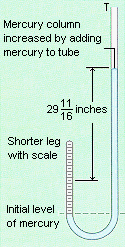Boyle studied what happened to the volume of the gas in the sealed end of the tube as he added mercury to the open end.

Boyle noticed that the product of the pressure times the volume for any measurement in this table was equal to the product of the pressure times the volume for any other measurement, within experimental error.

P1V1 = P2V2

This expression, or its equivalent,is now known as Boyle's Law.

 Practice Problem 3:Calculate the pressure in atmospheres in a motorcycle engine at the end of the compression stroke.  Assume that at the start of the stroke, the pressure of the mixture of gasoline and air in the cylinder is 745.8 mm Hg and the volume of each cylinder is 246.8 mL.  Assume that the volume of the cylinder is 24.2 mL at the end of the compression stroke. Click here to check your answer to Practice Problem 3. Click here to see a solution to Practice Problem 3.Amonton's Law

Toward the end of the 1600s, the French physicist Guillaume Amontons built a thermometer based on the fact that the pressure of a gas is directly proportional to its temperature. The relationship between the pressure and the temperature of a gas is therefore known as Amontons' law.

P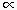T

Amontons' law explains why car manufacturers recommend adjusting the pressure of your tires before you start on a trip. The flexing of the tire as you drive inevitably raises the temperature of the air in the tire. When this happens, the pressure of the gas inside the tires increases.

Amontons' law can be demonstrated with the apparatus shown in the figure below, which consists of a pressure gauge connected to a metal sphere of constant volume, which is immersed in solutions that have different temperatures.The apparatus for demonstrating Amonton's law consists of .

The following data were obtained with this apparatus.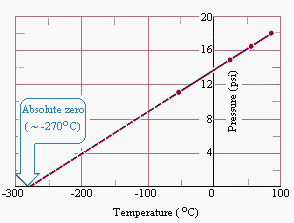In 1779 Joseph Lambert proposed a definition for absolute zero on the temperature scale that was based on the straight-line relationship between the temperature and pressure of a gas shown in the figure above.

He defined absolute zero as the temperature at which the pressure of a gas becomes zero when a plot of pressure versus temperature for a gas is extrapolated. The pressure of a gas approaches zero when the temperature is about -270°C. When more accurate measurements are made, the pressure of a gas extrapolates to zero when the temperature is -273.15°C. Absolute zero on the Celsius scale is therefore -273.15°C.

The relationship between temperature and pressure can be greatly simplified by converting the temperatures from the Celsius to the Kelvin scale.

TK = ToC + 273.15

When this is done, a plot of the temperature versus the pressure of a gas gives a straight line that passes through the origin. Any two points along the line therefore fit the following equation.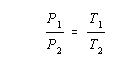It is important to remember that this equation is only valid if the temperatures are converted from the Celsius to the Kelvin scale before calculations are done.

 Practice Problem 4:Assume that the pressure in the tires of your car is 32 lb/in2 at 20°C.  What is the pressure when the gas in these tires heats up to a temperature of 40°C? Click here to check your answer to Practice Problem 4. Click here to see a solution to Practice Problem 4.Charles' Law

On 5 June 1783, Joseph and Etienne Montgolfier used a fire to inflate a spherical balloon about 30 feet in diameter that traveled about a mile and one-half before it came back to earth. News of this remarkable achievement spread throughout France, and Jacques-Alexandre-Cesar Charles immediately tried to duplicate this performance. As a result of his work with balloons, Charles noticed that the volume of a gas is directly proportional to its temperature.

VT

This relationship between the temperature and volume of a gas, which became known as Charles' law, provides an explanation of how hot-air balloons work. Ever since the third century B.C., it has been known that an object floats when it weighs less than the fluid it displaces. If a gas expands when heated, then a given weight of hot air occupies a larger volume than the same weight of cold air. Hot air is therefore less dense than cold air. Once the air in a balloon gets hot enough, the net weight of the balloon plus this hot air is less than the weight of an equivalent volume of cold air, and the balloon starts to rise. When the gas in the balloon is allowed to cool, the balloon returns to the ground.

Charles' law can be demonstrated with the apparatus shown in the figure below. A 30-mL syringe and a thermometer are inserted through a rubber stopper into a flask that has been cooled to 0ºC. The ice bath is then removed and the flask is immersed in a warm-water bath. The gas in the flask expands as it warms, slowly pushing the piston out of the syringe. The total volume of the gas in the system is equal to the volume of the flask plus the volume of the syringe.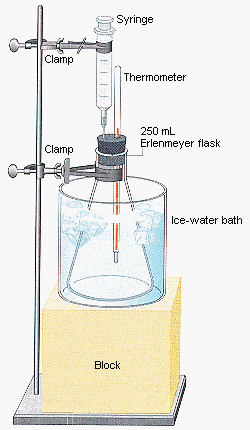The figure below shows a plot of the typical data obtained from this experiment.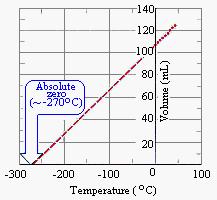This graph provides us with another way of defining absolute zero on the temperature scale. Absolute zero is the temperature at which the volume of a gas becomes zero when the a plot of the volume versus temperature for a gas are extrapolated. As expected, the value of absolute zero obtained by extrapolating the data is essentially the same as the value obtained from the graph of pressure versus temperature in the preceding section. Absolute zero can therefore be more accurately defined as the temperature at which the pressure and the volume of a gas extrapolate to zero.

A plot of the volume versus the temperature of a gas (when the temperatures obtained are converted from Celsius to the Kelvin scale) becomes a straight line that passes through the origin. Any two points along this line can therefore be used to construct the following equation, which is known as Charles' law.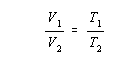Before using this equation, it is important to remember that temperatures must be converted from ºC to K.

 Practice Problem 5:Assume that the volume of a balloon filled with H2 is 1.00 L at 25°C.  Calculate the volume of the balloon when it is cooled to -78°C in a low-temperature bath made by adding dry ice to acetone. Click here to check your answer to Practice Problem 5. Click here to see a solution to Practice Problem 5.Gay-Lussac's Law

Joseph Louis Gay-Lussac (1778-1850) began his career in 1801 by very carefully showing the validity of Charles' law for a number of different gases. Gay-Lussac's most important contributions to the study of gases, however, were experiments he performed on the ratio of the volumes of gases involved in a chemical reaction.

Gay-Lussac studied the volume of gases consumed or produced in a chemical reaction because he was interested in the reaction between hydrogen and oxygen to form water. He argued that measurements of the weights of hydrogen and oxygen consumed in this reaction could be influenced by the moisture present in the reaction flask, but this moisture would not affect the volumes of hydrogen and oxygen gases consumed in the reaction.

Much to his surprise, Gay-Lussac found that 199.89 parts by volume of hydrogen were consumed for every 100 parts by volume of oxygen. Thus, hydrogen and oxygen seemed to combine in a simple 2:1 ratio by volume.

 hydrogen + oxygenwater 2 volumes 1 volume

Gay-Lussac found similar whole-number ratios for the reaction between other pairs of gases. The compound we now know as hydrogen chloride (HCl) combined with ammonia (NH3) in a simple 1:1 ratio by volume:

 hydrogen chloride + ammoniaammonium chloride 1 volume 1 volume

Carbon monoxide combined with oxygen in a 2:1 ratio by volume:

 carbon monoxide + oxygencarbon dioxide 2 volumes 1 volume

Gay-Lussac obtained similar results when he analyzed the volumes of gases given off when compounds decomposed. Ammonia, for example, decomposed to give three times as much hydrogen by volume as nitrogen:

 ammonianitrogen + hydrogen 1 volume 3 volumes

On 31 December 1808, Gay-Lussac announced his law of combining volumes to a meeting of the Societ Philomatique in Paris. At that time, he summarized the law as follows: Gases combine among themselves in very simple proportions. Today, Gay-Lussac's law is stated as follows: The ratio of the volumes of gases consumed or produced in a chemical reaction is equal to the ratio of simple whole numbers.

 Practice Problem 6:Use the following balanced chemical equations to explain the results of Gay-Lussac's experiments: 2 H2(g) + O2(g)2H2O(g) HCl(g) + NH3(g)NH4Cl(s) 2 CO(g) + O2(g)2 CO2(g) 2 NH3(g)N2 + 3 H2(g) Click here to check your answer to Practice Problem 6.Avogadro's Hypothesis

Gay-Lussac's law of combining volumes was announced only a few years after John Dalton proposed his atomic theory. The link between these two ideas was first recognized by the Italian physicist Amadeo Avogadro three years later, in 1811. Avogadro argued that Gay-Lussac's law of combining volumes could be explained by assuming that equal volumes of different gases collected under similar conditions contain the same number of particles.

HCl and NH3 therefore combine in a 1:1 ratio by volume because one molecule of HCl is consumed for every molecule of NH3 in this reaction and equal volumes of these gases contain the same number of molecules.

 NH3(g) + HCl(g)NH4Cl(s)

Anyone who has blown up a balloon should accept the notion that the volume of a gas is proportional to the number of particles in the gas.

Vn

The more air you add to a balloon, the bigger it gets. Unfortunately this example does not test Avogadro's hypothesis that equal volumes of different gases contain the same number of particles. The best way to probe the validity of this hypothesis is to measure the number of molecules in a given volume of different gases, which can be done with the apparatus shown in the figure below.A small hole is drilled through the plunger of a 50-mL plastic syringe. The plunger is then pushed into the syringe and the syringe is sealed with a syringe cap. The plunger is then pulled out of the syringe until the volume reads 50 mL and a nail is inserted through the hole in the plunger so that the plunger is not sucked back into the barrel of the syringe. The "empty" syringe is then weighed, the syringe is filled with 50 mL of a gas, and the syringe is reweighed. The difference between these measurements is the mass of 50 mL of the gas.

The results of experiments with six gases are given in the table below.

Experimental Data for the Mass of 50-mL Samples of Different Gases

 Compound Mass of 50 mL of Gas (g) Molecular Weight of Gas Number of Gas Molecules H2 0.005 2.02 1 x 1021 N2 0.055 28.01 1.2 x 1021 O2 0.061 32.00 1.1 x 1021 CO2 0.088 44.01 1.2 x 1021 C4H10 0.111 58.12 1.15 x 1021 CCl2F2 0.228 120.91 1.14 x 1021

The number of molecules in a 50-mL sample of any one of these gases can be calculated from the mass of the sample, the molecular weight of the gas, and the number of molecules in a mole. Consider the following calculation of the number of H2 molecules in 50 mL of hydrogen gas, for example.The last column in the table above summarizes the results obtained when this calculation is repeated for each gas. The number of significant figures in the answer changes from one calculation to the next. But the number of molecules in each sample is the same, within experimental error. We therefore conclude that equal volumes of different gases collected under the same conditions of temperature and pressure do in fact contain the same number of particles.The Ideal Gas Equation

Gases can described in terms of four variables: pressure (P), volume (V), temperature (T), and the amount of gas (n). There are five relationships between pairs of these variables in which two of the variables were allowed to cahnge while the other two were held constant.

 Pn (T and V constant) Boyle's law: P1/V (T and n constant) Amontons' law: PT (V and n constant) Charles' law: VT (P and n constant) Avogadro's hypothesis: Vn (P and T constant)

Each of these relationships is a special case of a more general relationship known as the ideal gas equation.

PV = nRT

In this equation, R is a proportionality constant known as the ideal gas constant and T is the absolute temperature. The value of R depends on the units used to express the four variables P, V, n, and T. By convention, most chemists use the following set of units.

 P: atmospheres T: kelvin V: liters n: moles
 Practice Problem 7:Calculate the value of the ideal gas constant, R, if exactly 1 mole of an ideal gas occupies a volume of 22.414 liters at 0°C and 1 atmosphere pressure. Click here to check your answer to Practice Problem 7. Click here to see a solution to Practice Problem 7.The ideal gas equation can be used to predict the value of any one of the variables that describe a gas from known values of the other three.

 Practice Problem 8: Many gases are available for use inthe laboratory in compressed gas cylinders, in which they are stored at high pressures. Let's calculate the mass of O2 that can be stored at 21ºC and 170 atm in a cylinder with a volume of 60.0 L. Click here to check your answer to Practice Problem 8. Click here to see a solution to Practice Problem 8.

The key to solving ideal gas problems often involves recognizing what is known and deciding how to use this information.

 Practice Problem 9:Let's calculate the mass of the air in a hot-air balloon that has a volume of 4.00 x 105 liters when the temperature of the gas is 30ºC and the presure is 748 mmHg. Let's assume the average molar mass of air is 29.0 grams per mole. Click here to check your answer to Practice Problem 9. Click here to see a solution to Practice Problem 9.

The ideal gas equation can be applied to problems that don't seem to ask for one of the variables in this equation.

 Practice Problem 10:Let's calculate the molecular weight of butane if 0.5813 gram of this gas fills a 250.0-mL flask at a temperature of 24.4ºC and a pressure of 742.6 mmHg. Click here to check your answer to Practice Problem 10. Click here to see a solution to Practice Problem 10.

The ideal gas equation can even be used to solve problems that don't seem to contain enough information.

 Practice Problem 11:Let's calculate the density in grams per liter of O2 gas at 0ºC and 1.00 atm. Click here to check your answer to Practice Problem 11. Click here to see a solution to Practice Problem 11.

Gas law problems often ask you to predict what happens when one or more changes are made in the variables that describe the gas. There are two ways of working these problems. A powerful approach is based on the fact that the ideal gas constant is in fact a constant.

We start by solving the ideal gas equation for the ideal gas constant.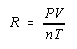We then note that the ratio of PV/nT at any time must be equal to this ratio at any other time.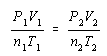We then substitute the known values of pressure, temperature, volume, and amount of gas into this equation and solve for the appropriate unknown. This approach has two advantages. First, only one equation has to be remembered. Second, it can be used to handle problems in which more than one variable changes at a time.Dalton's Law of Partial Pressures

The CRC Handbook of Chemistry and Physics describes the atmosphere as 78.084% N2, 20.946% O2, 0.934% Ar, and 0.033% CO2 by volume when the water vapor has been removed. What image does this description evoke in your mind? Do you believe that only 20.463% of the room in which you are sitting contains O2? Or do you believe that the atmosphere in your room is a more or less homogeneous mixture of these gases?

Gases expand to fill their containers. The volume of O2 in your room is therefore the same as the volume of N2. (Both gases expand to fill the room.) When we describe the atmosphere as 20.946% O2 by volume, we mean that the volume of the atmosphere would shrink by 20.946% if the O2 is removed.

What about the pressure of the different gases in your room? Is the pressure of the O2 in the atmosphere the same as the pressure of the N2? We can answer this question by rearranging the ideal gas equation as follows.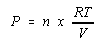According to this equation, the pressure of a gas is proportional to the number of moles of gas, if the temperature and volume are held constant. Because the temperature and volume of the O2 and N2 in the atmosphere are the same, the pressure of each gas must be proportional to the number of the moles of the gas. Because there is more N2 in the atmosphere than O2, the contribution to the total pressure of the atmosphere from N2 is larger than the contribution from O2.

John Dalton was the first to recognize that the total pressure of a mixture of gases is the sum of the contributions of the individual components of the mixture. By convention, the part of the total pressure of a mixture that results from one component is called the partial pressure of that component. Dalton's law of partial pressures states that the total pressure of a mixture of gases is the sum of the partial pressures of the various components.

 Practice Problem 12:Calculate the total pressure of a mixture that contains 1.00 g H2 and 1.00 g of He in a 5.00 L container at 21° C. Click here to check your answer to Practice Problem 12. Click here to see a solution to Practice Problem 12.

Dalton derived the law of partial pressures from his work on the amount of water vapor that could be absorbed by air at different temperatures. It is therefore fitting that this law is used most often to correct for the amount of water vapor picked up when a gas is collected by displacing water. Suppose, for example, that we want to collect a sample of O2 prepared by heating potassium chlorate until it decomposes.

 2 KClO3(s)2 KCl(s) + 3 O2(g)

The gas given off in this reaction can be collected by filling a flask with water, inverting the flask in a trough, and then letting the gas bubble into the flask as shown in the figure below.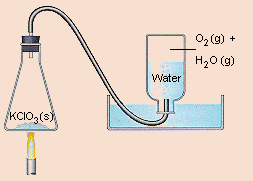Because some of the water in the flask will evaporate during the experiment, the gas that collects in this flask is going to be a mixture of O2 and water vapor. The total pressure of this gas is the sum of the partial pressures of these two components.

PT = Poxygen + Pwater

The total pressure of this mixture must be equal to atmospheric pressure. (If it was any greater, the gas would push water out of the container. If it was any less, water would be forced into the container.) If we had some way to estimate the partial pressure of the water in this system, we could therefore calculate the partial pressure of the oxygen gas.

By convention, the partial pressure of the gas that collects in a closed container above a liquid is known as the vapor pressure of the liquid. If we know the temperature at which a gas is collected by displacing water, and we assume that the gas is saturated with water vapor at this temperature, we can calculate the partial pressure of the gas by subtracting the vapor pressure of water from the total pressure of the mixture of gases collected in the experiment.

 Practice Problem 13:Let's calculate the number of grams of O2 that can be collected by displacing water from a 250-mL flask at 21ºC and 746.2 mmHg. Click here to check your answer to Practice Problem 13. Click here to see a solution to Practice Problem 13.